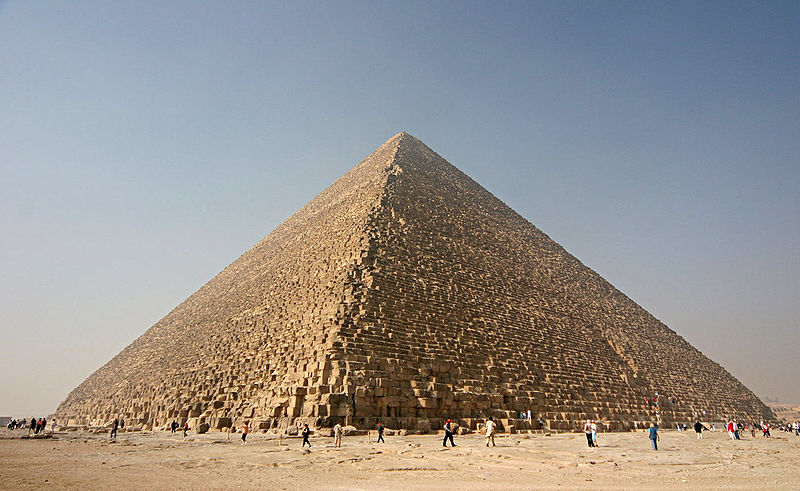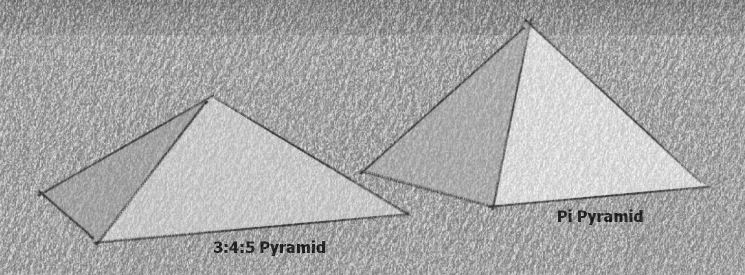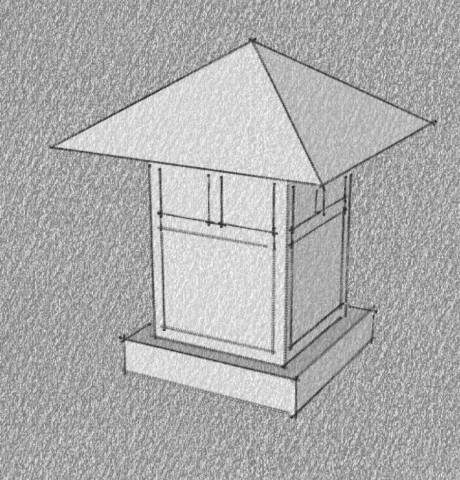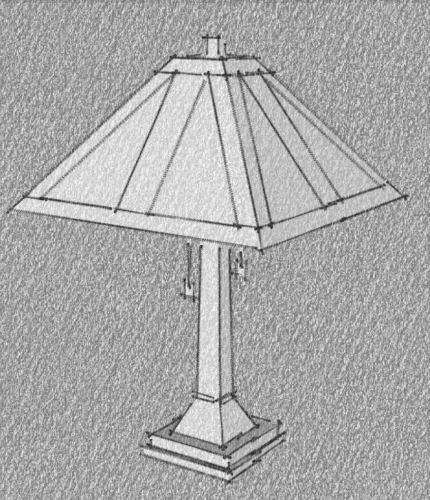# 02.02.03: Pyramid

## ExampleSource: Wikipedia

## Where to Use

Use when desiring to introduce the quintessential Egyptian architectural element, the Pyramid, into a design.

## Abstraction

Two square pyramid designs used in Egyptian architecture have medial triangles given by:

• Pi pyramid (h:b) = (4:pi) and perimeter = 2pi * height
• Golden pyramid (b:h:a) = (1 : sqrt(phi) : phi) which is a geometric progression of the sides
• 3:4:5 pyramid (b:h:a) = (3 : 4 : 5) which is an arithmetic progression of the sidesNote that the ratio of dimensions in a golden pyramid is very close to those of a pi pyramid.Mission-style lamps often use a pyramidal shape for the light shade:## Related Forms

Related forms are